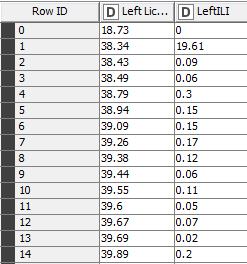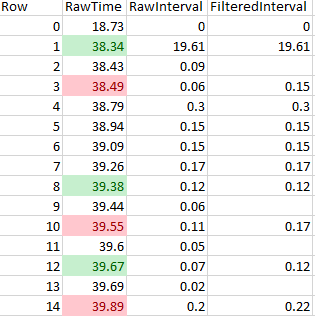# Filtering intervals between timestamp data in a column and recalculating intervals

Hi folks,

I have a basic understanding of KNIME but I’ve gotten a bit stuck on how to implement the following workflow:

I have a column which contains sequential timestamp data in seconds. I have managed to figure out how to calculate the intervals between each timestamp and output it as a new column:What I would like to do is to then filter my data so that when a time interval that is less than a set filter value (say interval less than or equal to 0.09 but not 0) is encountered, that it removes that timestamp and uses the next sequential timestamp in the next row to recalculate a new interval. If that new interval is greater than the set filter value, it outputs that value then moves on and it checks the next pair in the next row.

I have an excel sheet of the same data that shows what I’m trying to achieve using a knime workflow:I’ve highlighted some of the cells in the above example for clarity. When the workflow encounters a raw interval below the filter interval cutoff value, I’d like it it to skip that timestamp and have it calculate the interval without, it using the next timestamp (in the example, the red cell value minus the green cell value). When it encounters several intervals in a row that are below the filter value, like Rows 11-13, I’d like to have the workflow calculate intervals as indicated.

I’ve been spinning my wheels for a bit, so any insights would be very much appreciated.

Thanks!

Ken

Thanks!

Hi @KT_Wakabayashi1. Use the Rule-based Row Filter to filter out the RawInterval column values that is less than the cutoff point. Let’s say the cutoff point is 0.1, then the rule you set might look like this:

\$RawInterval\$ < 0.1 => TRUE

1. Then, to get the FiteredInterval column, calculate the new intervals the way you did for the raw intervals, using the new table you got from the previous step 1.

Hope that helps!

3 Likes

Interesting problem. Could you please copy & paste here in text format your solved sample (2nd excel snapshot) or upload a csv file with it, so that we can play with it and provide you with a workflow solution? Thanks in advance.

Best
Ael

1 Like

Thanks for your reply. Unfortunately if I try it that way, I end up removing all sequential and contiguous instances of intervals that fall below the cutoff point, such as all of the rows from rows 11,12 and 13, instead of only removing 11 and 13. Hence my getting stuck and asking for help on the forumExample for Forum.xlsx (17.2 KB)

Hi @aworker,

I have attached the solved sample as an .xlsx file, as I was unable to upload a csv file to the forums. I calculated these values manually, so I have not included the formulas in the cells of the uploaded sample.

Thank you!

Thanks for the data. Amazingly, the logic of your formula is much more involved than it sounds due to the fact that the current new row value to generate depends on previous row values but also on previous newly calculated row result. This is the kind of things where one easily straggle to get a solution in KNIME.

I could have implemented this using a recursive loop and setting a couple of variables in the body of the loop but eventually decided to solve it using a Java snippet. It is not for nothing that the -Java Snippet- node also exists!

So please find here below the piece of Java code and the workflow that implements a possible solution to your problem:

``````// Your custom variables:
Double cumulative = 0.0;
``````

and

``````// Enter your code here:
if ( cumulative == 0.0 && (c_RawInterval > 0.09 || c_RawInterval == 0.0)){
cumulative = 0.0;
out_result = c_RawInterval;
}
else {
if ( cumulative == 0.0){
cumulative = c_RawInterval;
// Missing Value. Replace by next instruction if numerical value is required : out_result = 0.0;
}
else {
if ( cumulative + c_RawInterval > 0.09){
out_result = cumulative + c_RawInterval;
cumulative = 0.0;
}
else {
cumulative = cumulative + c_RawInterval;
// Missing Value. Replace by next instruction if numerical value is required : out_result = 0.0;
}
}
}
out_cumulative = cumulative;

// expression end
``````

Since two new columns are created in this solution, one with the final result and a second one with an intermediate result to better understand what the code is doing, the following -Java Snippet" configuration of output columns is needed:

The workflow with the -Java Snippet- node is available from here:

One can see in previous snapshot that the expected column result called “FilteredInterval (>0.09)” and the one generated by the -Java Snippet- node called “result” are the same. The “cumulative” column is there just to show how intermediate results were calculated.

Hope it helps.

Best,

3 Likes

This topic was automatically closed 7 days after the last reply. New replies are no longer allowed.